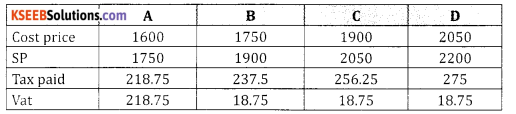# 2nd PUC Basic Maths Question Bank Chapter 12 Sales Tax and Value Added Tax Ex 12.2

Students can Download Basic Maths Exercise 12.2 Questions and Answers, Notes Pdf, 2nd PUC Basic Maths Question Bank with Answers helps you to revise the complete Karnataka State Board Syllabus and score more marks in your examinations.

## Karnataka 2nd PUC Basic Maths Question Bank Chapter 12 Sales Tax and Value Added Tax Ex 12.2

Part – A

2nd PUC Basic Maths Sales Tax and Value Added Tax Ex 12.2 Two or Three Marks Questions with Answers

Question 1.
A shopkeeper purchase an article for ₹ 9,000 and sell it to a customer for 10,500. If the VAT rate is 4%. Find the VAT paid by the shopkeeper?
Solution:
Given S.P = 10,500, C.P. = 9000, Vat = ?, = 4%
Vat = $$\frac{\text { Rate }}{100}$$ (SP-CP) = $$\frac{ 4 }{100}$$ (10,500 – 9000) =\frac{\text { Rate }}{100}[//latex] × 1500 = 60
∴ Vat paid by shopkeeper = ₹ 60.Question 2.
A shopkeeper purchased an electric iron of ₹ 1000 at 8% VAT from the whole saler and sell it to customer of ₹ 1400 at 8% VAT.
Solution:
Given
S.P = ₹ 1400, C.P = ₹ 1000, 8% = Vat rate, Vat = ?
Vat = $$\frac{\text { Rate }}{100}$$ (SP-CP)
Vat = $$\frac{8}{100}$$ (1400 – 1000)
= $$\frac{8 \times 400}{100}$$ = 32
∴ Vat paid = ₹ 32.

Question 3.
A shopkeeper bought a TV at a discount of 30% of the listed price of ₹ 24,000. The shopkeeper offer a discount of 10% of the listed price to the customer. If the VAT is 10%. Find (i) the amount paid by the customer. (ii) the VAT to be paid by the shopkeeper.
Solution:
Selling price of the shopkeeper = 70% of 24,000
= $$\frac{70}{100}$$ × 24,000 = 16,800
100 Selling price of the customer = 90% of 24,000
= $$\frac{90}{100}$$ × 24000 = 21600
Amount paid by the customer = 21600 + 10% of 21600
= 21600 + $$\frac{10}{100}$$ × 21600 = 21600 + 2160 = 23760
∴ Vat is 10% (21600 – 16800)
= $$\frac{10}{100}$$ × 4800 = ₹ 480Question 4.
Mohan a owner of a departmental store purchased an article of ₹ 1,500 at 4% VAT and sell it at ₹ 1,700 to the customer at 4% VAT. How much amount did the shopkeeper deposit to the government as VAT.
Solution:
Vat paid by the customer = 4% of 1700
= $$\frac{4}{100}$$ × 1700= ₹ 68
Vat paid by shopkeeper = 4% (1700 – 1500)
= $$\frac{4}{100}$$ × 200 = ₹ 78.

Question 5.
Sanju a owner of jewellary shop purchased a earring of ₹ 2,000 at 12% VAT and sells it at 2,300 to the customer Radhika. If Radhika also pays 12% VAT to the shopkeeper how much did the shopkeeper deposit to the government as VAT?
Solution:
Vat paid by shopkeeper = 12% of (2300 – 2000)
= $$\frac{12}{100}$$ × (300)
= ₹ 36.

Question 6.
‘A’ is manufacture of electric iron. The cost price of each electric iron in ₹ 1,600. He sells to ‘B’and ‘B’sells to ‘C’ and ‘C’ sells to ‘C’ sells to ‘D’ the retailer. The tax rate is 12.5% and the profit is ₹ 150 at each stage of the selling chain. Find the (i) the total amount of VAT and (ii) the amount that the purchased will have to pay.
Solution:Amount that the retailer will have to pay is
= 2200 + 275 = 2475
Total amount of vat = 21875 + 3(18.75)
= 218.75 + 56.25 = ₹ 275.Part – B

2nd PUC Basic Maths Sales Tax and Value Added Tax Ex 12.2 Two or Three Marks Questions with Answers

Question 1.
A furniture dealer sold furniture for ₹ 21000 & added 5% sales tax to the quoted price. The customer agrees to buy it for ₹ 21,000 including sales tax. Find the discount he received (July – 2015)
Solution:
Discount = 5% of 21,000
= $$\frac{5}{100}$$ × 21000 = 1050.
∴ Customer received discount = ₹ 31050
Amount to be paid = 21000 + 5% of 21000
= 21000 + $$\frac{5}{100}$$ × 21000 = 21000 + 1050 = 22050.Question 2.
The owner of a departmental store purchased an article of F1500 at 4% vat & set it at 1700 is the consumer at 4% vat. How much amount did the shopkeeper deposit to the government as vat?
Solution:
(P.V. Model paper 3)
The shopkeeper purchase price = M.P + 4% of M.P.
= 1500 + 4% of 1500
= 1500 + ( $$\frac{4}{100}$$ × 1500 ) = 1500= 1500+60 = 1560
Selling price of the shopkeeper = 1700 + 4% of 1700
= 1700 + ( $$\frac{4}{100}$$ × 1700) = 1700 + 68 = 1768.
∴ Total vat deposited to government
= 1768 – 1560 = ₹ 208.